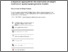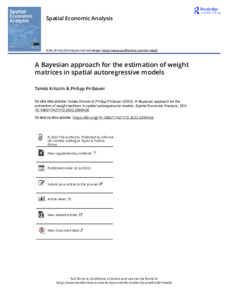# A Bayesian approach for the estimation of weight matrices in spatial autoregressive models

& Piribauer, P. (2022). A Bayesian approach for the estimation of weight matrices in spatial autoregressive models. Spatial Economic Analysis 1-20. 10.1080/17421772.2022.2095426.Preview Text A Bayesian approach for the estimation of weight matrices in spatial autoregressive models.pdf - Published Version Available under License Creative Commons Attribution. Download (1MB) | Preview

## Abstract

We develop a Bayesian approach to estimate weight matrices in spatial autoregressive (or spatial lag) models. Datasets in regional economic literature are typically characterized by a limited number of time periods (Formula presented.) relative to spatial units (Formula presented.). When the spatial weight matrix is subject to estimation severe problems of over-parametrization are likely. To make estimation feasible, our approach focusses on spatial weight matrices which are binary prior to row-standardization. We discuss the use of hierarchical priors which impose sparsity in the spatial weight matrix. Monte Carlo simulations show that these priors perform very well where the number of unknown parameters is large relative to the observations. The virtues of our approach are demonstrated using global data from the early phase of the COVID-19 pandemic.

Item Type: Article Bayesian Markov chain Monte Carlo (MCMC) estimation; COVID-19 pandemic; estimation of spatial weight matrix; Monte Carlo simulations; spatial econometric model Biodiversity and Natural Resources (BNR)Biodiversity and Natural Resources (BNR) > Integrated Biosphere Futures (IBF) Luke Kirwan 28 Jul 2022 10:43 28 Jul 2022 10:43 https://pure.iiasa.ac.at/18132View Item